Courses

# NCERT Solutions - Units & Dimensions Class 11 Notes | EduRev

## UPSC : NCERT Solutions - Units & Dimensions Class 11 Notes | EduRev

The document NCERT Solutions - Units & Dimensions Class 11 Notes | EduRev is a part of the UPSC Course Physics Class 11.
All you need of UPSC at this link: UPSC

Q.2.1.
Fill in the blanks
(a) The volume of a cube of side 1 cm is equal to ______ m3
(b) The surface area of a solid cylinder of radius 2.0 cm and height 10.0 cm is equal to ______ (mm)2
(c) A vehicle moving with a speed of 18 km h–1covers ______ m in 1 s
(d) The relative density of lead is 11.3. Its density is ______ g cm–3or ______ kg m–3.

Ans.
(a) 1 cm = (1/100)m
Volume of the cube = 1 cm
But, 1 cm3 = 1 cm × 1 cm × 1 cm
= (1/100) m × (1/100) m × (1/100)m
∴ 1 cm3 = 10–6 m3
Hence, the volume of a cube of side 1 cm is equal to 10–6 m3.
(b) The total surface area of a cylinder of radius r and height h is
S = 2πr (r h).
Given that,
r = 2 cm = 2 × 1 cm
= 2 × 10 mm = 20 mm
h = 10 cm = 10 × 10 mm
= 100 mm
∴ S = 2 × 3.14 × 20 × (20+100)
= 15072 = 1.5 × 104 mm2
(c) Using the conversion,
1 km/h = (5/18) m/s
18 km/h = 18 × (5/18) = 5 m/s

Therefore, distance can be obtained using the relation:
Distance = Speed × Time = 5 × 1 = 5 m
Hence, the vehicle covers 5 m in 1 s.
(d) Relative density of a substance is given by the relation,
Relative density = (Density of substance/Density of water)
Density of water = 1 g/cm3
Density of lead = Relative density of lead x Density of water = 11.3 × 1 = 11.3 g/cm3
Again,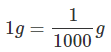1 cm3 = 10–6 m3
1 g/cm3 = (10-3/10-6)kg/m3
= 103 kg/m3
∴ 11.3 g/cm3 = 11.3 × 103 kg/m3

Q.2.2. Fill in the blanks by suitable conversion of units:
(a) 1 kg m2s–2= ______ g cm2 s–2
(b) 1 m =______ ly
(c) 3.0 m s–2=______ km h–2
(d) G= 6.67 × 10–11 N m2 (kg)–2=______ (cm)3s–2 g–1.

Ans.
(a) 1 kg = 103 g
1 m2 = 104 cm2
1 kg m2 s–2 = 1 kg × 1 m2 × 1 s–2
=103 g × 104 cm× 1 s–2 = 107 g cm2 s–2

(b) Light year is the total distance travelled by light in one year.
1 ly = Speed of light × One year
= (3 × 108 m/s) × (365 × 24 × 60 × 60 s)
= 9.46 × 1015 m
∴ 1 m = (1/9.46 × 1015)
= 1.057 × 10-16 ly

(c) 1 m = 10–3 km
Again,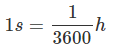1 s–1 = 3600 h–1
1 s–2 = (3600)2 h–2
∴ 3 m s–2 = (3 × 10–3 km) × ((3600)2 h–2)
= 3.88 × 104 km h–2

(d) 1 N = 1 kg m s–2
1 kg = 10–3 g–1
1 m3 = 106 cm3
∴ 6.67 × 10–11 N m2 kg–2
= 6.67 × 10–11 × (1 kg m s–2) (1 m2) (1 s–2)
= 6.67 × 10–11 × (1 kg × 1 m3 × 1 s–2)
= 6.67 × 10–11 × (10–3 g–1) × (106 cm3) × (1 s–2)
= 6.67 × 10–8 cm3 s–2 g–1

Q.2.3. A calorie is a unit of heat or energy and it equals about 4.2 J where 1 J = 1 kg m2s–2. Suppose we employ a system of units in which the unit of mass equals α kg, the unit of length equals β m, the unit of time is γ s. Show that a calorie has a magnitude 4.2 α–1 β–2 γin terms of the new units.
Ans. Given that,
1 calorie = 4.2 (1 kg) (1 m2) (1 s–2)
New unit of mass = α kg
Hence, in terms of the new unit, 1 kg = (1/α) = α-1
In terms of the new unit of length,
1 m = 1/β = β-1 or 1 m2 = β-2
And, in terms of the new unit of time,
1s = 1/γ = γ-1
1s2 = γ-2
1s-2 = γ2
∴ 1 calorie = 4.2 (1 α–1) (1 β–2) (1 γ2)
= 4.2 α–1 β–2 γ2

Q.2.4. Explain this statement clearly:
“To call a dimensional quantity ‘large’ or ‘small’ is meaningless without specifying a standard for comparison”. In view of this, reframe the following statements wherever necessary:
(a) Atoms are very small objects
(b) A jet plane moves with great speed
(c) The mass of Jupiter is very large
(d) The air inside this room contains a large number of molecules
(e) A proton is much more massive than an electron
(f) The speed of sound is much smaller than the speed of light.

Ans. The given statement is true because a dimensionless quantity may be large or small in comparison to some standard reference.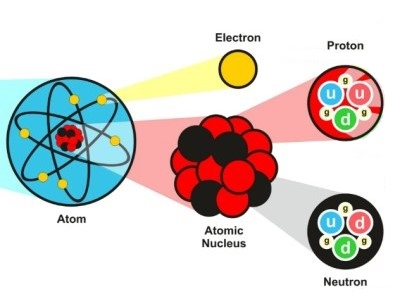Size Comparision

For example, the coefficient of friction is dimensionless. The coefficient of sliding friction is greater than the coefficient of rolling friction, but less than static friction.
(a) An atom is a very small object in comparison to a soccer ball.
(b) A jet plane moves with a speed greater than that of a bicycle.
(c) Mass of Jupiter is very large as compared to the mass of a cricket ball.
(d) The air inside this room contains a large number of molecules as compared to that present in a geometry box.
(e) A proton is more massive than an electron.
(f) Speed of sound is less than the speed of light.

Q.2.5. A new unit of length is chosen such that the speed of light in vacuum is unity. What is the distance between the Sun and the Earth in terms of the new unit if light takes 8 min and 20 s to cover this distance?
Ans. Distance between the Sun and the Earth:
= Speed of light × Time taken by light to cover the distance
Given that in the new unit, speed of light = 1 unit
Time taken, t = 8 min 20 s = 500 s
∴ Distance between the Sun and the Earth = 1 × 500 = 500 units

Q.2.6. Which of the following is the most precise device for measuring length:
(a) a vernier callipers with 20 divisions on the sliding scale
(b) a screw gauge of pitch 1 mm and 100 divisions on the circular scale
(c) an optical instrument that can measure length to within a wavelength of light ?

Ans. A device with minimum count is the most suitable to measure length.
(a) Least count of vernier caliper
= 1 standard division (SD) – 1 vernier division (VD)
= 1 - (9/10) = (1/10) = 0.01 cm

(b) Least count of screw gauge = (Pitch/Number of divisions)
= (1/1000) = 0.001 cm

(c) Least count of an optical device = Wavelength of light ∼ 10–5 cm = 0.00001 cm
Hence, it can be inferred that an optical instrument is the most suitable device to measure length.

Q.2.7. A student measures the thickness of a human hair by looking at it through a microscope of magnification 100. He makes 20 observations and finds that the average width of the hair in the field of view of the microscope is 3.5 mm. What is the estimate on the thickness of hair?
Ans. Magnification of the microscope = 100
Average width of the hair in the field of view of the microscope = 3.5 mm
∴ Actual thickness of the hair is 3.5/100 = 0.035 mm.

(a) You are given a thread and a metre scale. How will you estimate the diameter of the thread?
(b) A screw gauge has a pitch of 1.0 mm and 200 divisions on the circular scale. Do you think it is possible to increase the accuracy of the screw gauge arbitrarily by increasing the number of divisions on the circular scale?
(c) The mean diameter of a thin brass rod is to be measured by vernier callipers. Why is a set of 100 measurements of the diameter expected to yield a more reliable estimate than a set of 5 measurements only?
Ans.
(a) Wrap the thread on a uniform smooth rod in such a way that the coils thus formed are very close to each other. Measure the length of the thread using a metre scale. The diameter of the thread is given by the relation,
Diameter = Length of thread/Number of turns

(b) It is not possible to increase the accuracy of a screw gauge by increasing the number of divisions of the circular scale. Increasing the number divisions of the circular scale will increase its accuracy to a certain extent only.

(c) A set of 100 measurements is more reliable than a set of 5 measurements because random errors involved in the former are very less as compared to the latter.

Q.2.9. The photograph of a house occupies an area of 1.75 cm2 on a 35 mm slide. The slide is projected on to a screen, and the area of the house on the screen is 1.55 m2. What is the linear magnification of the projector-screen arrangement?
Ans. Area of the house on the slide = 1.75 cm2
Area of the image of the house formed on the screen = 1.55 m
= 1.55 × 104 cm2
Arial magnification, ma = (Area of image/Area of object) = (1.55/1.75) × 104
∴ Linear magnifications,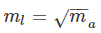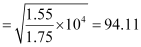Q.2.10. State the number of significant figures in the following:
(a) 0.007 m2
(b) 2.64 × 1024 kg
(c) 0.2370 g cm–3
(d) 6.320 J
(e) 6.032 N m–2
(f) 0.0006032 m2
Ans.
(a)  1
The given quantity is 0.007 m2.
If the number is less than one, then all zeros on the right of the decimal point (but left to the first non-zero) are insignificant. This means that here, two zeros after the decimal are not significant. Hence, only 7 is a significant figure in this quantity.

(b) 3
The given quantity is 2.64 × 1024 kg.
Here, the power of 10 is irrelevant for the determination of significant figures. Hence, all digits i.e., 2, 6 and 4 are significant figures.

(c) 4
The given quantity is 0.2370 g cm–3.
For a number with decimals, the trailing zeroes are significant. Hence, besides digits 2, 3 and 7, 0 that appears after the decimal point is also a significant figure.

(d) 4
The given quantity is 6.320 J.
For a number with decimals, the trailing zeroes are significant. Hence, all four digits appearing in the given quantity are significant figures.

(e) 4
The given quantity is 6.032 Nm–2.
All zeroes between two non-zero digits are always significant.

(f) 4
The given quantity is 0.0006032 m2.
If the number is less than one, then the zeroes on the right of the decimal point (but left to the first non-zero) are insignificant. Hence, all three zeroes appearing before 6 are not significant figures. All zeros between two non-zero digits are always significant. Hence, the remaining four digits are significant figures.

Q.2.11. The length, breadth and thickness of a rectangular sheet of metal are 4.234 m, 1.005 m, and 2.01 cm respectively. Give the area and volume of the sheet to correct significant figures.
Ans.
Length of sheet, = 4.234 m
Breadth of sheet, = 1.005 m
Thickness of sheet, = 2.01 cm = 0.0201 m
The given table lists the respective significant figures: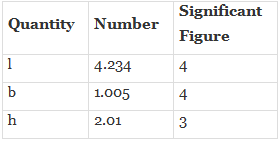Hence, area and volume both must have least significant figures i.e., 3.
Surface area of the sheet = 2 ((l × b) +(× h)+(h × l))
= 2((4.234 × 1.005)+ (1.005 × 0.0201)+(0.0201 × 4.234))
= 2 (4.25517 + 0.02620 + 0.08510)
= 2 × 4.360
= 8.72 m2
Volume of the sheet = × b × h
= 4.234 × 1.005 × 0.0201
= 0.0855 m3
This number has only 3 significant figures i.e., 8, 5, and 5.

Q. 2.12. The mass of a box measured by a grocer’s balance is 2.300 kg. Two gold pieces of masses 20.15 g and 20.17 g are added to the box. What is (a) the total mass of the box, (b) the difference in the masses of the pieces to correct significant figures?
Ans.
Mass of grocer’s box = 2.300 kg
Mass of gold piece I = 20.15 g = 0.02015 kg
Mass of gold piece II = 20.17 g = 0.02017 kg
(a) Total mass of the box = 2.3 0.02015 0.02017 = 2.34032 kg
In addition, the final result should retain as many decimal places as there are in the number with the least decimal places. Hence, the total mass of the box is 2.3 kg.
(b) Difference in masses = 20.17 – 20.15 = 0.02 g
In subtraction, the final result should retain as many decimal places as there are in the number with the least decimal places.

Q. 2.13. A physical quantity is related to four observables a, b, c and as follows: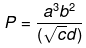The percentage errors of measurement in a, b, and d are 1%, 3%, 4% and 2%, respectively. What is the percentage error in the quantity P? If the value of calculated using the above relation turns out to be 3.763, to what value should you round off the result?
Ans.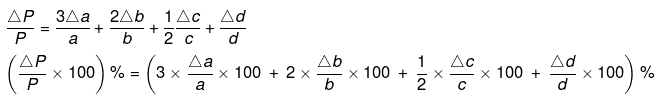3 × 1 + 2 × 3 + (1/2) × 4 + 2
= 3 + 6 + 2 + 2 = 13%
Percentage error in P = 13 %
Value of is given as 3.763.
By rounding off the given value to the first decimal place, we get P = 3.8.

Q. 2.14: A book with many printing errors contains four different formulas for the displacement of a particle undergoing a certain periodic motion:
(a) y = a sin(2πt/T)
(b) = sin vt
(c) y = (a/T) sin(t/a)
(d) y = (a√2) (sin(2πt/T) + cos(2πt/T))

(= maximum displacement of the particle, = speed of the particle. = time-period of motion). Rule out the wrong formulas on dimensional grounds.
Ans.
(a) Correct
y = a sin(2πt/T)
Dimension of y = M0 L1 T0
Dimension of a = M0 L1 T0
Dimension of sin (2πt/T)= M0 L0 T0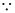Dimension of L.H.S = Dimension of R.H.S
Hence, the given formula is dimensionally correct.

(b) Incorrect
y = a sin vt
Dimension of y = M0 L1 T0
Dimension of a = M0 L1 T0
Dimension of vt = M0 L1 T–1 × M0 L0 T= M0 L1 T0
But the argument of the trigonometric function must be dimensionless, which is not so in the given case. Hence, the given formula is dimensionally incorrect.

(c) Incorrect
y = (a/T) sin(t/a)
Dimension of y = M0L1T0
Dimension of  a/T = M0L1T–1
Dimension of  t/a = M0 L–1 T1
But the argument of the trigonometric function must be dimensionless, which is not so in the given case. Hence, the formula is dimensionally incorrect.

(d) Correct
y = (a√2)(sin2π(t/T) + cos2π(t/T)
Dimension of y = M0 L1 T0
Dimension of a = M0 L1 T0
Dimension of  t/T = M0 L0 T0
Since the argument of the trigonometric function must be dimensionless (which is true in the given case), the dimensions of y and a are the same. Hence, the given formula is dimensionally correct.

Q.2.15. A famous relation in physics relates ‘moving mass’ to the ‘rest mass’ m0 of a particle in terms of its speed and the speed of light, c. (This relation first arose as a consequence of special relativity due to Albert Einstein). A boy recalls the relation almost correctly but forgets where to put the constant c. He writes:
m = (m0/1-v2)1/2
Ans.
Given the relation,
m = (m0/1-v2)1/2
Dimension of m = M1 L0 T0
Dimension of m= M1 L0 T0
Dimension of v = M0 L1 T–1
Dimension of v2 = M0 L2 T–2
Dimension of c = M0 L1 T–1
The given formula will be dimensionally correct only when the dimension of L.H.S is the same as that of R.H.S. This is only possible when the factor,(1-v2)1/2 is dimensionless i.e., (1 – v2) is dimensionless. This is only possible if v2 is divided by c2. Hence, the correct relation is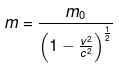Q.2.16. The unit of length convenient on the atomic scale is known as an angstrom and is denoted by Å : 1 Å = 10-10m. The size of a hydrogen atom is about 0.5Å . what is the total atomic volume in m3 of a mole of hydrogen atoms?
Ans.
Radius of hydrogen atom, r = 0.5 Å = 0.5 × 10–10 m
Volume of hydrogen atom = (4/3)πr3
= (4/3 × 22/7) × (0.5 × 10-10)3
= 0.524 × 10-30m3
1 mole of hydrogen contains 6.023 × 1023 hydrogen atoms.
∴ Volume of 1 mole of hydrogen atoms = 6.023 × 1023 × 0.524 × 10–30
= 3.16 × 10–7 m3

Q.2.17. One mole of an ideal gas at standard temperature and pressure occupies 22.4 L (molar volume). What is the ratio of molar volume to the atomic volume of a mole of hydrogen? (Take the size of hydrogen molecule to be about 1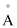). Why is this ratio so large?
Ans. Radius of hydrogen atom, r = 0.5= 0.5 × 10–10 m
Volume of hydrogen atom = (4/3)πr3
= (4/3 × 22/7) × (0.5 x 10-10)3
= 0.524 x 10-30 m3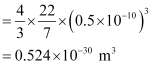Now, 1 mole of hydrogen contains 6.023 × 1023 hydrogen atoms.
∴ Volume of 1 mole of hydrogen atoms, Va = 6.023 × 1023 × 0.524 × 10–30
= 3.16 × 10–7 m3
Molar volume of 1 mole of hydrogen atoms at STP,
Vm = 22.4 L = 22.4 × 10–3 m3
∴ Vm/Va = (22.4 × 10-3/3.16 × 10-7) = 7.08 × 104
Hence, the molar volume is 7.08 × 104 times higher than the atomic volume. For this reason, the inter-atomic separation in hydrogen gas is much larger than the size of a hydrogen atom.

Q.2.18. Explain this common observation clearly : If you look out of the window of a fast moving train, the nearby trees, houses etc. seem to move rapidly in a direction opposite to the train’s motion, but the distant objects (hill tops, the Moon, the stars etc.) seem to be stationary. (In fact, since you are aware that you are moving, these distant objects seem to move with you).
Ans. Line of sight is defined as an imaginary line joining an object and an observer’s eye. When we observe nearby stationary objects such as trees, houses, etc. while sitting in a moving train, they appear to move rapidly in the opposite direction because the line of sight changes very rapidly. On the other hand, distant objects such as trees, stars, etc. appear stationary because of the large distance. As a result, the line of sight does not change its direction rapidly.

Q.2.19. The principle of ‘parallax’ in section 2.3.1 is used in the determination of distances of very distant stars. The baseline AB is the line joining the Earth’s two locations six months apart in its orbit around the Sun. That is, the baseline is about the diameter of the Earth’s orbit ≈ 3 × 1011m. However, even the nearest stars are so distant that with such a long baseline, they show parallax only of the order of 1” (second) of arc or so. A parsec is a convenient unit of length on the astronomical scale. It is the distance of an object that will show a parallax of 1” (second) of arc from opposite ends of a baseline equal to the distance from the Earth to the Sun. How much is a parsec in terms of meters?
Ans. Diameter of Earth’s orbit = 3 × 1011 m
Radius of Earth’s orbit, r = 1.5 × 1011 m
Let the distance parallax angle be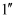= 4.847 × 10–6 rad.
Let the distance of the star be D.
Parsec is defined as the distance at which the average radius of the Earth’s orbit subtends an angle of.
∴ We have θ = (r/D)
D = r/θ = (1.5 × 1011/4.847 × 10-6)
= 0.309 × 10-6 ≈ 3.09 × 1016 m
Hence, 1 parsec ≈ 3.09 × 1016 m.

Q.2.20: The nearest star to our solar system is 4.29 light years away. How much is this distance in terms of parsecs? How much parallax would this star (named Alpha Centauri) show when viewed from two locations of the Earth six months apart in its orbit around the Sun?
Ans.
Distance of the star from the solar system = 4.29 ly
1 light year is the distance travelled by light in one year.
1 light year = Speed of light × 1 year
= 3 × 108 × 365 × 24 × 60 × 60 = 94608 × 1011 m
∴ 4.29 ly = 405868.32 × 1011 m1 parsec = 3.08 × 1016 m
∴ 4.29 ly = (405868.32 × 1011)/(3.08 × 1016) = 1.32 parsec
Using the relation,
θ = d/ D
where,
Diameter of Earth`s orbit d = 3 × 1011 m
Distance of the star from the Earth, D = 405868.32 × 1011 m
∴ θ = (3 × 1011)/(405868.32 × 1011)
But, 1 sec = 4.85 × 10–6 rad
= (7.39 × 10-6/4.85 × 10-6) = 1.52"

Q.2.21. Precise measurements of physical quantities are a need of science. For example, to ascertain the speed of an aircraft, one must have an accurate method to find its positions at closely separated instants of time. This was the actual motivation behind the discovery of radar in World War II. Think of different examples in modern science where precise measurements of length, time, mass etc. are needed. Also, wherever you can, give a quantitative idea of the precision needed.
Ans. It is indeed very true that precise measurements of physical quantities are essential for the development of science. For example, ultra-shot laser pulses (time interval ∼ 10–15 s) are used to measure time intervals in several physical and chemical processes.
X-ray spectroscopy is used to determine the inter-atomic separation or inter-planer spacing.
The development of mass spectrometer makes it possible to measure the mass of atoms precisely.

Q.2.22. Just as precise measurements are necessary in science, it is equally important to be able to make rough estimates of quantities using rudimentary ideas and common observations. Think of ways by which you can estimate the following (where an estimate is difficult to obtain, try to get an upper bound on the quantity):
(a) The total mass of rain-bearing clouds over India during the Monsoon
(b) The mass of an elephant
(c) The wind speed during a storm
(e) The number of air molecules in your classroom.
Ans.
(a) During monsoons, a metrologist records about 215 cm of rainfall in India i.e., the height of water column, h = 215 cm = 2.15 m
Area of country, A = 3.3 × 1012 m2
Hence, volume of rain water, = A × h
= 7.09 × 1012 m3
Density of water, ρ = 1 × 103 kg m–3
Hence, mass of rain water = ρ × V
= 7.09 × 1015 kg
Hence, the total mass of rain-bearing clouds over India is approximately 7.09 × 1015 kg.

(b) Consider a ship of known base area floating in the sea. Measure its depth in sea (say d1).
Volume of water displaced by the ship, Vb = A d1
Now, move an elephant on the ship and measure the depth of the ship (d2) in this case.
Volume of water displaced by the ship with the elephant on board, Vbe= Ad2
Density of water = D
Mass of elephant = AD (d2d1)

(c) Wind speed during a storm can be measured by an anemometer. As wind blows, it rotates. The rotation made by the anemometer in one second gives the value of wind speed.

(d) Area of the head surface carrying hair = A
With the help of a screw gauge, the diameter and hence, the radius of a hair can be determined. Let it be r.
∴ Area of one hair = πr2
Number of strands of hair ≈ (Total surface area/Area of one hair π) = A/r2

(e) Let the volume of the room be V.
One mole of air at NTP occupies 22.4 l
i.e., 22.4 × 10–3 m3 volume.
Number of molecules in one mole = 6.023 × 1023
∴ Number of molecules in room of volume V
= (6.023 × 1023 / 22.4 × 10-3) × V
= 134.915 × 1026 V
= 1.35 × 1028 V

Q. 2.23. The Sun is a hot plasma (ionized matter) with its inner core at a temperature exceeding 107 K, and its outer surface at a temperature of about 6000 K. At these high temperatures, no substance remains in a solid or liquid phase. In what range do you expect the mass density of the Sun to be, in the range of densities of solids and liquids or gases? Check if your guess is correct from the following data: mass of the Sun = 2.0 × 1030 kg, radius of the Sun = 7.0 × 108 m.
Ans. Mass of the Sun, M = 2.0 × 1030 kg
Radius of the Sun, R = 7.0 × 108 m
Volume of the Sun, V = (4/3) πR3
= (4/3) × (22/7) × (7.0 × 108)3
= (88/21) × 343 × 1024
= 1437.3 × 1024 m3
Density of the Sun = Mass/Volume = (2.0 × 1030/1437.3 × 1024) ∼ 1.4 × 103 kg/m5
The density of the Sun is in the density range of solids and liquids. This high density is attributed to the intense gravitational attraction of the inner layers on the outer layer of the Sun.

Q.2.24. When the planet Jupiter is at a distance of 824.7 million kilometers from the Earth, its angular diameter is measured to be 35.72" of arc. Calculate the diameter of Jupiter.
Ans.
Distance of Jupiter from the Earth,
D = 824.7 × 106 km = 824.7 × 109 m
Angular diameter =35.72" = 35.72 × 4.874 × 10-6 rad
Diameter of Jupiter = d
Using the relation,
θ = d/D
d = θD = 824.7 × 109 × 35.72 × 4.872 × 10-6
= 143520.76 × 103
= 1.435 × 105 km

Q.2.25. A man walking briskly in rain with speed must slant his umbrella forward making an angle θ with the vertical. A student derives the following relation between θ and v: tan θ = v and checks that the relation has a correct limit: as v0, θ → 0, as expected. (We are assuming there is no strong wind and that the rain falls vertically for a stationary man). Do you think this relation can be correct? If not, guess the correct relation.
Ans. Incorrect;
on dimensional ground
The relation is tan θ = v.
Dimension of R.H.S = M0 L1 T–1
Dimension of L.H.S = M0 L0 T0
(∴ The trigonometric function is considered to be a dimensionless quantity)
Dimension of R.H.S is not equal to the dimension of L.H.S. Hence, the given relation is not correct dimensionally.
To make the given relation correct, the R.H.S should also be dimensionless. One way to achieve this is by dividing the R.H.S by the speed of rainfall v'.
Therefore, the relation reduces to tan θ = v/v'.
This relation is dimensionally correct.

Q.2.26. It is claimed that two cesium clocks, if allowed to run for 100 years, free from any disturbance, may differ by only about 0.02 s. What does this imply for the accuracy of the standard cesium clock in measuring a time-interval of 1 s?
Ans. Difference in time of cesium clocks = 0.02 s
Time required for this difference = 100 years
= 100 × 365 × 24 × 60 × 60 = 3.15 × 109 s
In 3.15 × 109 s, the cesium clock shows a time difference of 0.02 s.
In 1s, the clock will show a time difference of (0.02/3.15 × 109)s.
Hence, the accuracy of a standard cesium clock in measuring a time interval of 1 s is (3.15 × 109/0.02)
= 157.5 × 10s ≈ 1.5 × 1011 s.

Q.2.27. Estimate the average mass density of a sodium atom assuming its size to be about 2.5. (Use the known values of Avogadro’s number and the atomic mass of sodium). Compare it with the density of sodium in its crystalline phase: 970 kg m–3. Are the two densities of the same order of magnitude? If so, why?
Ans. Diameter of sodium atom = Size of sodium atom = 2.5 Å
Radius of sodium atom, r = (1/2) × 2.5  Å = 1.25Å
= 1.25 × 10–10 m
Volume of sodium atom, V = (4/3) πr3
= (4/3) × 3.14 × (1.25 × 10-10)3
According to the Avogadro hypothesis, one mole of sodium contains 6.023 × 1023 atoms and has a mass of 23 g or 23 × 10–3 kg.
∴ Mass of one atom = (23 × 10-3/6.023 × 1023) kg

Density of sodium atom,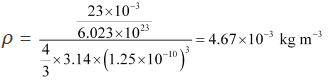It is given that the density of sodium in crystalline phase is 970 kg m–3.
Hence, the density of sodium atom and the density of sodium in its crystalline phase are not in the same order. This is because in solid phase, atoms are closely packed. Thus, the inter-atomic separation is very small in the crystalline phase.

Q.2.28. The unit of length convenient on the nuclear scale is a fermi: 1 f = 10–15 m. Nuclear sizes obey roughly the following empirical relation : r = r0A1/3
where is the radius of the nucleus, its mass number, and ris a constant equal to about, 1.2 f. Show that the rule implies that nuclear mass density is nearly constant for different nuclei. Estimate the mass density of sodium nucleus. Compare it with the average mass density of a sodium atom obtained in Exercise. 2.27.
Ans. Radius of nucleus r is given by the relation,
r = r0A1/3 ...(i)
r0= 1.2 f = 1.2 × 10–15 m
Volume of nucleus, V = 4/3 πr3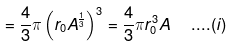Now, the mass of a nuclei M is equal to its mass number i.e.,
M = A amu = A × 1.66 × 10–27 kg
Density of nucleus,
ρ = Mass of nucleus/Volume of nucleus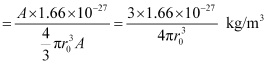This relation shows that nuclear mass depends only on constant r0. Hence, the nuclear mass densities of all nuclei are nearly the same.
Density of sodium nucleus is given by,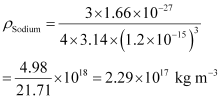Q.2.29. A LASER is a source of very intense, monochromatic, and unidirectional beam of light. These properties of a laser light can be exploited to measure long distances. The distance of the Moon from the Earth has been already determined very precisely using a laser as a source of light. A laser light beamed at the Moon takes 2.56 s to return after reflection at the Moon’s surface. How much is the radius of the lunar orbit around the Earth?
Ans. Time taken by the laser beam to return to Earth after reflection from the Moon = 2.56 s
Speed of light = 3 × 108 m/s
Time taken by the laser beam to reach Moon = (1/2) × 2.56 = 1.28s
Radius of the lunar orbit = Distance between the Earth and the Moon
= 1.28 × 3 × 108
= 3.84 × 108 m
= 3.84 × 105 km

Q.2.30. A SONAR (sound navigation and ranging) uses ultrasonic waves to detect and locate objects under water. In a submarine equipped with a SONAR the time delay between generation of a probe wave and the reception of its echo after reflection from an enemy submarine is found to be 77.0 s. What is the distance of the enemy submarine? (Speed of sound in water = 1450 m s–1).
Ans. Let the distance between the ship and the enemy submarine be ‘S’.
Speed of sound in water = 1450 m/s
Time lag between transmission and reception of Sonar waves = 77 s
In this time lag, sound waves travel a distance which is twice the distance between the ship and the submarine (2S).
Time taken for the sound to reach the submarine = (1/2) × 77 = 38.5 s
∴ Distance between the ship and the submarine (S) = 1450 × 38.5
= 55825 m
= 55.8 km

Q.2.31. The farthest objects in our Universe discovered by modern astronomers are so distant that light emitted by them takes billions of years to reach the Earth. These objects (known as quasars) have many puzzling features, which have not yet been satisfactorily explained. What is the distance in km of a quasar from which light takes 3.0 billion years to reach us?
Ans. Time taken by quasar light to reach Earth = 3 billion years
= 3 × 109 years
= 3 × 109 × 365 × 24 × 60 × 60 s
Speed of light = 3 × 108 m/s
Distance between the Earth and quasar
= (3 × 108) × (3 × 109 × 365 × 24 × 60 × 60)
= 283824 × 1020 m
= 2.8 × 1022 km

Q.2.32. It is a well known fact that during a total solar eclipse the disk of the moon almost completely covers the disk of the Sun. From this fact and from the information you can gather from examples 2.3 and 2.4, determine the approximate diameter of the moon.
Ans. The position of the Sun, Moon, and Earth during a lunar eclipse is shown in the given figure.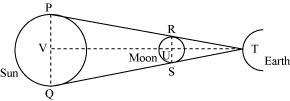Distance of the Moon from the Earth
= 3.84 × 108 m
Distance of the Sun from the Earth
= 1.496 × 1011 m
Diameter of the Sun = 1.39 × 109 m
It can be observed that ΔTRS and ΔTPQ are similar. Hence, it can be written as: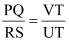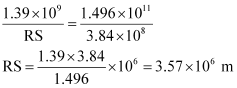Hence, the diameter of the Moon is 3.57 × 106 m.

Q.2.33. A great physicist of this century (P.A.M. Dirac) loved playing with numerical values of Fundamental constants of nature. This led him to an interesting observation. Dirac found that from the basic constants of atomic physics (c, e, mass of electron, mass of proton) and the gravitational constant G, he could arrive at a number with the dimension of time. Further, it was a very large number, its magnitude being close to the present estimate on the age of the universe (~15 billion years). From the table of fundamental constants in this book, try to see if you too can construct this number (or any other interesting number you can think of). If its coincidence with the age of the universe were significant, what would this imply for the constancy of fundamental constants?
Ans. One relation consists of some fundamental constants that give the age of the Universe by: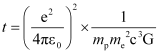Where,
t = Age of Universe
e = Charge of electrons = 1.6 ×10–19 C
ε0 = Absolute permittivity
mp = Mass of protons = 1.67 × 10–27 kg
mc = Mass of electrons = 9.1 × 10–31 kg
c = Speed of light = 3 × 108 m/s
G = Universal gravitational constant = 6.67 × 1011 Nm2 kg–2

Also,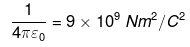Substituting these values in the equation, we get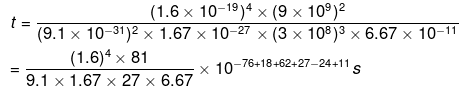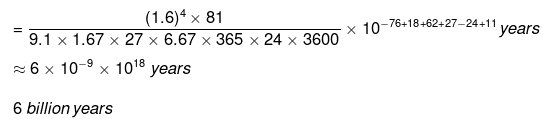Offer running on EduRev: Apply code STAYHOME200 to get INR 200 off on our premium plan EduRev Infinity!

## Physics Class 11

73 videos|313 docs|177 tests

,

,

,

,

,

,

,

,

,

,

,

,

,

,

,

,

,

,

,

,

,

;